# What is Java synchronization – Race condition problems solution

In this article, we will talk about what is java synchronization and how it can be used to solve race condition problems. We will use images of the java source code for our explanation. The samples illustrated in the article will be run in an Eclipse Integrated Development Environment specifically the Spring Tool Suite which is an open-source and free to acquire implementation of Eclipse. We will explain step by step how to create a race condition problem by running the program several times, and taking print screen images of the results. Then we will be adding changes to the program and showing the effect of the changes, and finally will create a complete program with all the necessary code to solve the problem.

## The multithread problem, the race condition:

Let us look at the Java MainClassA below. There is a variable called tally that is initialized to zero. Then the main method and the executeThreads method.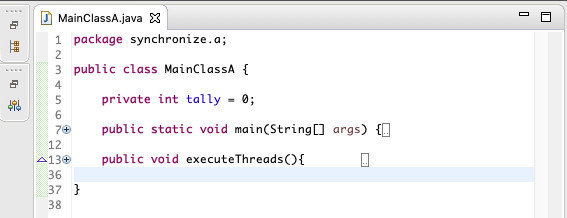In the image below we expanded the main method, as you can see, we instantiated MainClassA as an object called mcf. Then we call the executeThreads method from the mcf object.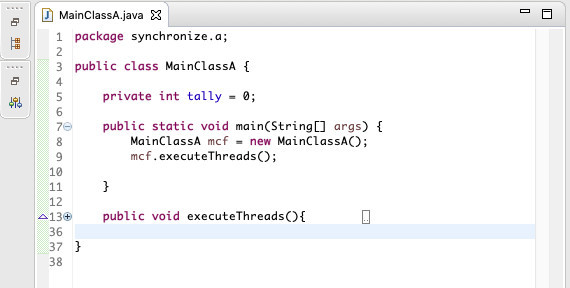Below we expanded the executeThreads method. In the method, we instantiated
the class Thread twice and on each instantiation, we passed a Runnable object as an anonymous class. Then we call the start method on each thread object.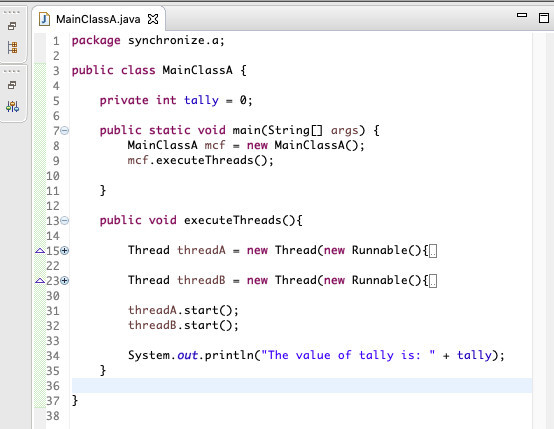Here we have the complete program, notice that the anonymous Runnable objects each do the same logic. The logic just runs a loop 15000 times and on each iteration, it increments the variable called tally. At the end of a program run, we should expect that tally will contain 30000 as its value.Look at the image below, notice at the right hand, the console after running the program. In the console, the program displayed a message that reads: The value of tally is: 0. This is wrong because it should have been 30000. We can see a problem with running these two threads that both of them change the value of the variable called tally. In this particular case what happened is that when the program  executes the main method and calls executeThreads method, the executeThreads method starts threadA and threadB, and immediately runs the next statement that displays the message “The value of tally is: 0”, so the statement that displays the message executed before the loops that change the value of tally.## Using the join method of the Thread class:

In the image below we can see that we added lines 34 and 35 and we are calling the join method of threadA and threadB. The join method will guarantee that the main method in MainClassA will wait for threadA and threadB to finish execution before displaying the message in the console. The join method throws an interruptedException so we need to handle the exception.To handle the InterruptedException we surround the two lines of code with a try/catch, see the block of code from lines 34 to 39. Now the program will not display tally with a value of zero, but we might still have other problems…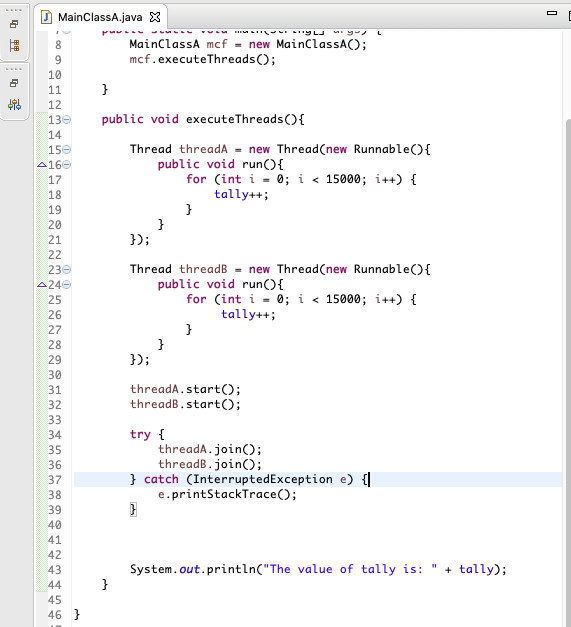As we mentioned above we still have problems. We ran the program multiple times and we kept getting erroneous values for the variable called tally. See below for an example, the variable called tally has a value of 15439. This is still wrong. We have a pretty good idea of what is happening. The main culprit is a statement in line 18 and the one in line 26:

tally++;

What happens is that the statement above internally works like this:

tally = tally + 1;

That above is 3 steps as follows:

1. Obtain the value of the variable called tally and put it in a working area.
2. Add one to the value of the variable called tally in the working area.
3. Move the value of the variable called tally from the working area into
the variable called tally in the main memory.

This might look like it is happening very fast, but in the computer CPU and where there are thousands of operations happening in one second, in between these three steps might happen many things. For instance, threadA obtains the value of tally which is 0, but before threadA has the time to add 1 to tally, threadB might obtain the value of tally and might even run the loop twice incrementing tally by 2 and updating tally in main memory with the value of 2. Meanwhile in threadA tally is 0 and threadA increments 0 to 1 and puts that value of 1 on top of the tally value of 2 in main memory. Tally should contain a value of 3 (one from threadA plus two from threadB) but it was erroneously updated with a value of 1. This is a race  condition and is a common problem in multithreaded programs.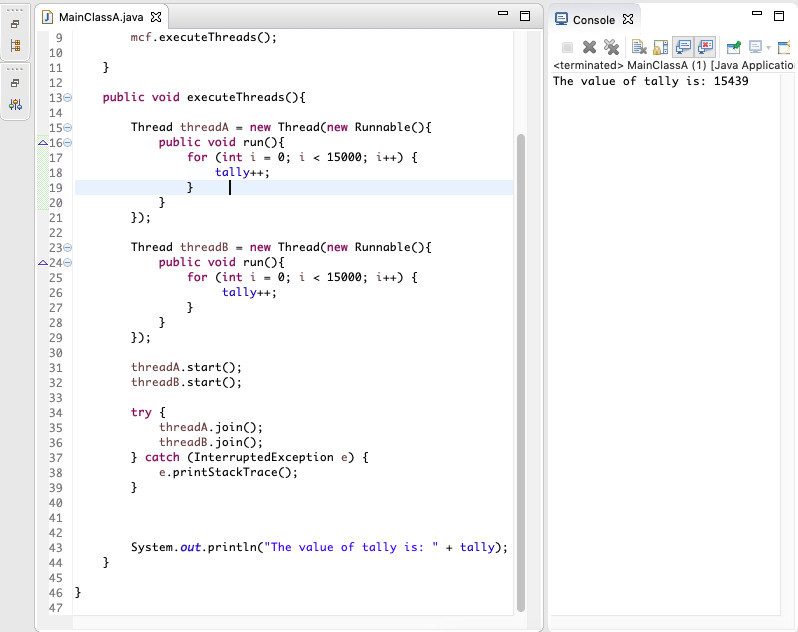## Isolating the code that we want to be synchronized:

To resolve this problem we are going to use a two-step solution, first, we will isolate the code or instruction that we want to synchronize. In this case, the  instruction is “ tally++;”. So we created a new method call add1, see lines 48 to 50 below. In lines 18 and 27 we commented out the code so that it does not run. Then in lines 19 and 28, we add calls to the new method add1. As mentioned this is a two-step process and this first step does not solve the issue.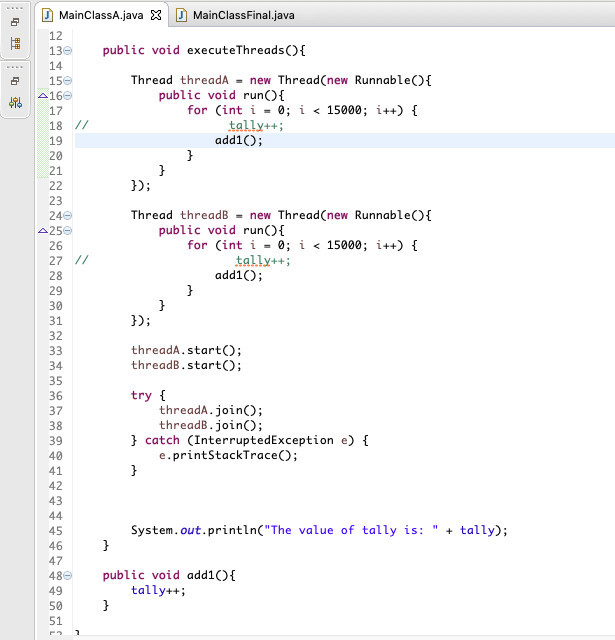As a test, we ran the code from step one of the process and we still get wrong values in the variable called tally, see the console message below that reads “The value of tally is: 22337”.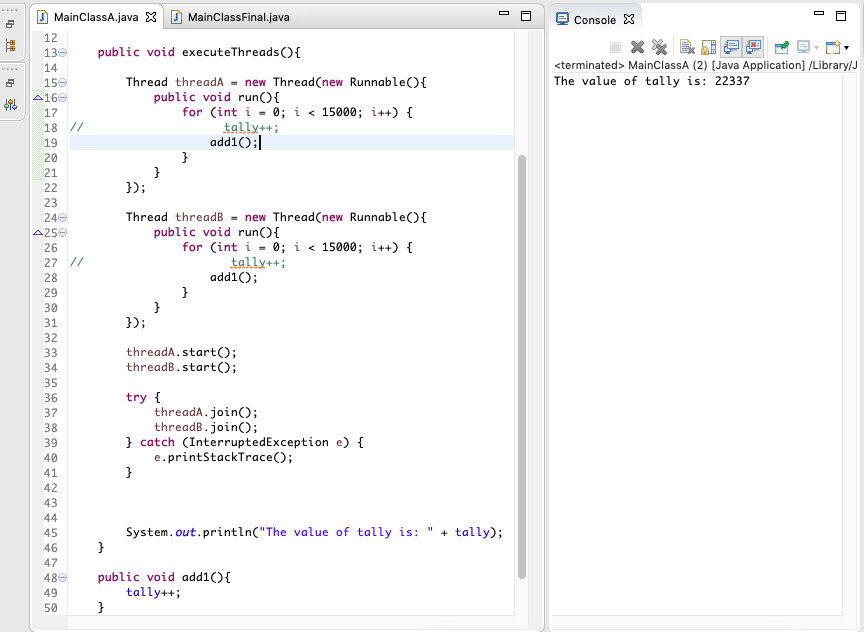In the image below see line 48 of the program. Notice we added the keyword synchronized. Then we ran the program and the result is a total value in the variable called tally of 30000, which is the correct value. We ran it many times and is always correct.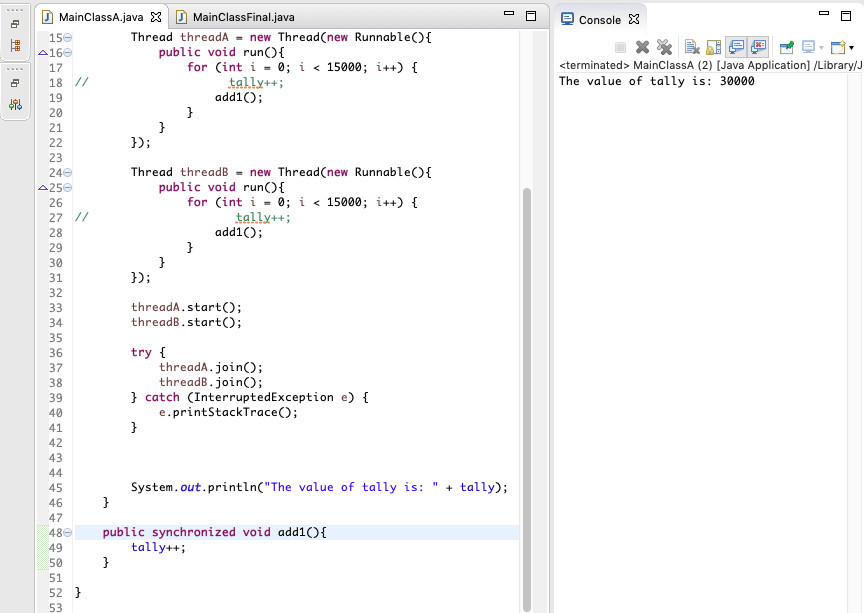## Synchronized how it works in a nutshell:

Another advantage of the keyword synchronized in Java is that if you use synchronized you no longer need to use the volatile keyword because synchronized keyword guarantees that the variables are visible for all threads all the time.

## Source code:

### Copy/paste the source code below in an Eclipse IDE, or your favorite IDE and format it for indentation.  In Eclipse on top menu select Source and then Correct Indentation.

package synchronize.last;

public class MainClassFinal {

private int tally = 0;

public static void main(String[] args) {
MainClassFinal mcf = new MainClassFinal();

}

public void run(){
for (int i = 0; i < 15000; i++) {
}
}
});

public void run(){
for (int i = 0; i < 15000; i++) {
}
}
});

try {
} catch (InterruptedException e) {
e.printStackTrace();
}

System.out.println(“The value of tally is: ” + tally);
}